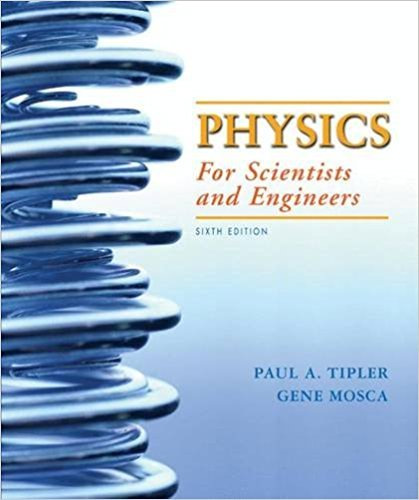×

# SPREADSHEET, ENGINEERING APPLICATION The initialISBN: 9781429201247 201

## Solution for problem 97 Chapter 8

Physics for Scientists and Engineers, | 6th Edition

• Textbook Solutions
• 2901 Step-by-step solutions solved by professors and subject experts
• Get 24/7 help from StudySoup virtual teaching assistantsPhysics for Scientists and Engineers, | 6th Edition

4 5 0 356 Reviews
17
5
Problem 97

SPREADSHEET, ENGINEERING APPLICATION The initial thrust-to-weight ratio of a rocket is where is the rockets thrust and the initial mass of the rocket, including the propellant. (a) For a rocket launched straight up from Earths surface, show that where is the initial acceleration of the rocket. For manned rocket flight, cannot be made much larger than 4 for the comfort and safety of the astronauts. (As the rocket lifts off, the astronauts will feel that their weight is equal to times their normal weight.) (b) Show that the final velocity of a rocket launched from Earths surface can, in terms of and (see 96), be written as where is the mass of the rocket (not including the spent propellant). (c) Using a spreadsheet program or graphing calculator, graph as a function of the mass ratio for and for values of the mass ratio from 2 to 10. (Note that the mass ratio cannot be less than 1.) (d) To lift a rocket into orbit, a final velocity after burnout of is needed. Calculate the mass ratio required of a single-stage rocket to do this, using the values of specific impulse and thrust ratio given in Part (b). For engineering reasons, it is difficult to make a rocket with a mass ratio much greater than 10. Can you see why multistage rockets are usually used to put payloads into orbit around Earth?

Step-by-Step Solution:
Step 1 of 3
Step 2 of 3

Step 3 of 3

##### ISBN: 9781429201247

Physics for Scientists and Engineers, was written by and is associated to the ISBN: 9781429201247. This full solution covers the following key subjects: . This expansive textbook survival guide covers 42 chapters, and 3686 solutions. Since the solution to 97 from 8 chapter was answered, more than 224 students have viewed the full step-by-step answer. The answer to “SPREADSHEET, ENGINEERING APPLICATION The initial thrust-to-weight ratio of a rocket is where is the rockets thrust and the initial mass of the rocket, including the propellant. (a) For a rocket launched straight up from Earths surface, show that where is the initial acceleration of the rocket. For manned rocket flight, cannot be made much larger than 4 for the comfort and safety of the astronauts. (As the rocket lifts off, the astronauts will feel that their weight is equal to times their normal weight.) (b) Show that the final velocity of a rocket launched from Earths surface can, in terms of and (see 96), be written as where is the mass of the rocket (not including the spent propellant). (c) Using a spreadsheet program or graphing calculator, graph as a function of the mass ratio for and for values of the mass ratio from 2 to 10. (Note that the mass ratio cannot be less than 1.) (d) To lift a rocket into orbit, a final velocity after burnout of is needed. Calculate the mass ratio required of a single-stage rocket to do this, using the values of specific impulse and thrust ratio given in Part (b). For engineering reasons, it is difficult to make a rocket with a mass ratio much greater than 10. Can you see why multistage rockets are usually used to put payloads into orbit around Earth?” is broken down into a number of easy to follow steps, and 231 words. The full step-by-step solution to problem: 97 from chapter: 8 was answered by , our top Physics solution expert on 01/02/18, 09:00PM. This textbook survival guide was created for the textbook: Physics for Scientists and Engineers,, edition: 6.

Unlock Textbook Solution

×